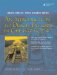# Member Access Specifiers

Thus far we have worked with class definition code and class implementation code. There is a third category of code as it relates to a given class. Client code is code that is outside the scope of the class but that uses objects of the class. Generally, client code #includes the header file that contains the class definition.

We now revisit the Fraction class, focusing on its member access specifiers. See Example 2.5.

Example 2.5. src/classes/fraction.h

 ```#ifndef _FRACTION_H_ #define _FRACTION_H_ #include using namespace std; class Fraction { public: void set(int numerator, int denominator); double toDouble() const; string toString() const; private: int m_Numerator; int m_Denominator; }; #endif ```

The member access specifiers, public, protected, and private, are used in a class definition to specify where in a program the affected members can be accessed. The following list provides an informal first approximation of the definitions of these three terms. Refinements are contained in footnotes.

• A public member can be accessed (using an object of the class) anywhere in a program that #includes the class definition file.

 public static members can be accessed without an object. We discuss this in Section 2.10.

• A protected member can be accessed inside the definition of a member function of its own class, or a member function of a derived class.

 We discuss derived classes in Chapter 6.

• A private member is only accessible by member functions of its own class.

 Private members are also accessible by friends of the class, which we discuss in Section 2.6.

## Accessibility versus Visibility

There is a subtle difference between accessibility and visibility. In order for a named item to be accessible, it must first be visible (in our scope). Not all visible items are accessible. Accessibility depends on member access specifiers: public/private/protected.

Example 2.6 shows client code that demonstrates visibility errors in a variety of ways. This example also focuses on block scope, which extends from an opening brace to a closing brace. A variable declared inside a block is visible and accessible only between its declaration and the closing brace. In the case of a function, the block that contains the function definition also includes the function's parameter list.

Example 2.6. src/classes/fraction-client.cpp

 ```#include "fraction.h" #include int main() { const int DASHES = 30; using namespace std; { <-- 1 int i; for(i = 0; i < DASHES; ++i) cout << "="; cout << endl; } // cout << "i = " << i << endl; <-- 2 Fraction f1, f2; f1.set(3, 4); f2.set(11,12); <-- 3 // f2.m_Numerator = 12; <-- 4 cout << "The first fraction is: " << f1.toString() << endl; cout << " The second fraction, expressed as a double is: " << f2.toDouble() << endl; return 0; } ```   (1)nested scopeinner block (2)errori no longer exists, so it is not visible in this scope. (3)set through a member function (4)errorm_Numerator is visible but not accessible.

Now we can describe the difference between struct and class in C++. Stroustrup defines a struct to be a class in which members are by default public, so that

```struct T { ...
```

means precisely

```class T {public: ...
```An Introduction to Design Patterns in C++ with Qt 4
ISBN: 0131879057
EAN: 2147483647
Year: 2004
Pages: 268